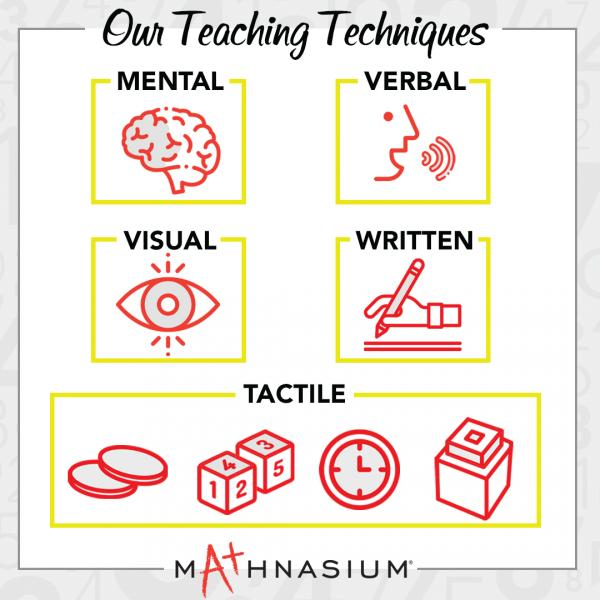* indicates a required field• phone number format invalid• email format invalidProblems detected, please review the form.
Privacy - Terms

We open for In-Center instruction and also offering online @home sessions

# News from Mathnasium of Cottage Grove

### Teaching Math in a Way That Makes Sense

Jul 5, 2021What is it about Mathnasium that helps students find success in math? The answer is simple: We teach for understanding. Instead of coaching students to rely on memorization, rote learning, or calculators, our highly trained instructors use multiple approaches, so children learn in ways that make sense to them. Because all students learn differently.

The Mathnasium Method™ is unique in that it employs five distinct modes to deliver our program to students, and lessons usually involve a combination of several of these modes. If a student doesn’t understand a concept one way, the instructor will use another mode until the student thinks, “Aha! I get it.” This deep understanding is the key to reaching their mathematical potential.

# Below are our five approaches and an explanation of how each one works.

Mental math is, quite simply, doing math in your head. When students develop confidence in their ability to solve problems mentally, it improves their self-esteem and willingness to explore math. It’s also often a much more efficient way to finding solutions. For example, adding 99 + 99 + 99, as shown here, is made easier by thinking of 100 x 3, then taking away 3.

Here are two more examples:

Problem:

What is 6½% of 200?

Mental Solution:

Think of 6½ for each 100. There are two 100s, and 6½ two times is 13. So 6½% of 200 is 13.

Problem:

What is ¾ of 20?

Mental Solution:

Developing confidence with the concept of halving and quartering allows you to recognize that ¼ of 20 is 5. 5 x 3 is 15. So ¾ of 20 is 15.

These thought processes are far more efficient than traditional methods, and they develop higher levels of problem-solving skills in our students.

# Visual cues help students focus on the question.

Visual learning focuses on what we can see. Students who gravitate toward visual learning do much better with visual cues that help them understand a concept. For this reason, a significant number of Mathnasium worksheets contain pictures, figures, graphs, scaffolding, and other visual prompts. These help students make important mathematical connections.

# Comprehension often comes through verbal interaction.

Some students respond more favorably to the spoken word. This is known as verbal, or linguistic, learning. Multiple forms of verbal interaction guide a student to understanding, and “direct teaching” is a good example. Instructors walk students step by step through a lesson in their Learning Plan, checking frequently with the student to ensure the student understands each part of the concept before moving to the next step.

“Socratic questioning” is another verbal mode in which instructors help students to understand a concept by asking the student questions. This is the method Socrates used with his students. By answering questions, students have to make sure their logic is sound.

Finally, instructors ask students to explain how they got their answers. That verbal exchange encourages students to think about the mathematical process rather than simply calculate.

# Touching and holding objects can make all the difference.

Tactile learning, also known as kinesthetic learning, involves touching, manipulating, or physically experiencing a concept for better understanding and retention. For students who respond best to these techniques, we use manipulatives (coins, dice, cards, scales, clocks, fraction circles, etc.) to guide them through the thought process. Each manipulative targets a particular concept that needs to be mastered:

• Coins not only provide students exposure to currency but are critical for understanding strategic grouping and proportional reasoning.
• Dice and cards can be used to help a student practice basic math facts.
• Clocks help a student tell time, calculate elapsed time, and even provide a foundation for multiplication.
• Fraction circles provide essential reinforcement to the relative value of fractions, an understanding invaluable to a student’s future math success.

# Solving problems through written methods.

Written learning takes place through the reading and writing of text, which is how some learners absorb and retain the most information. To address their needs, Mathnasium teaches algorithms for addition, subtraction, multiplication, and division. Our written materials allow students to experience the most efficient, accessible algorithm for any given topic. We build Number Sense through these algorithms as well. This process enables students to start to look at problems, see multiple ways to attack them, and choose the best, most efficient one.

# We can identify which techniques will work best for your child.

Mathnasium’s teaching techniques are tailored to the individual learning styles and needs of each child. They are specifically designed to help fill in knowledge gaps and build a solid mathematical foundation that enables students to feel competent and confident in learning and doing math. We invite you to stop by our center and ask the center director to demonstrate some of our techniques with your child.